# tharwan.de

## How much storage does the "Energiewende" need? Roughly?

To get some rought estimates we will at first decide to ignore a few things outright:

1. that Germany is not alone but part of a European wide grid
2. Sectorcoupling, i.e. that you can store energy in form of heat, or switch your heating between electric power and burning fossile fuels directly

There are much more sophisticated tools which include these like: PyPSA

Our data source is transparency.entsoe.eu (be sure to select country not bidding zone which is the default) where you can download the data once you created an account. We use the data for load and production for all of 2016. The data comes in 15min time resolution. This Jupyter notebook can also be accessed here.

In :
%matplotlib notebook
import numpy as np
import pandas
import matplotlib.pylab as plt
import datetime
import warnings

In :
#setup a date parser so we can aggregate the data later
dateparse = lambda x: pandas.datetime.strptime(x[:16], '%d.%m.%Y %H:%M')

#import data with pandas
parse_dates=,date_parser=dateparse,index_col=0)
generation = pandas.read_csv("Actual Generation per Production Type_201601010000-201701010000.csv",
parse_dates=,date_parser=dateparse,index_col=1)


We will make another assumption concerning power from biomass. Not only does it appear the growth period for biomass in Germany is over, there are also good reasons to not push it any further. In essence, their efficiency is below those of wind and solar, and its use needs to be limited in order to be sustainable.

We will therefore include the generation from biomass into the load, which we assume stays constant over all calculations, while we will scale the production (e.g. wind and solar) up to different values.

In :
#print(generation.columns)

wind_year = generation.filter(regex="Wind.*Actual Aggregated.*").sum().sum()
solar_year = generation.filter(regex="Solar.*Actual Aggregated.*").sum().sum()
bio_year = generation.filter(regex="Biomass.*Actual Aggregated.*").sum().sum()
other_renew = generation.filter(regex="(Waste|Geothermal|Marine|renewable|Hydro Run|Hydro Water).*Actual Aggregated.*").sum().sum()
fossile = generation.filter(regex="(Fossil|Nuclear).*Actual Aggregated.*").sum().sum()

tmp = np.array([wind_year,solar_year,bio_year,other_renew,fossile])/1E6*0.25
labels = ["wind","solar","bio","other","fossile+nuclear"]
plt.figure(figsize=(6,2))
ax = plt.subplot()
left = 0
for idx in range(len(tmp)):
plt.barh(0,tmp[idx],1,left,label=labels[idx])
left += tmp[idx]
plt.legend(loc=7)
plt.tight_layout()
plt.xlim((0,450))
ax.set_yticklabels(())
ax.set_yticks(())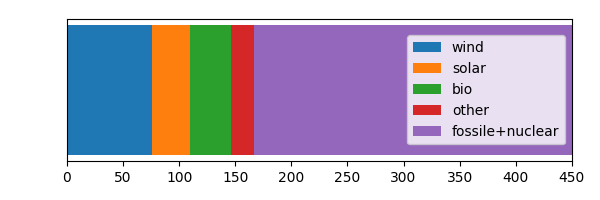Out:
[]
In :
# next we grab the parts that we acually need
# there are some nans in the data mostly because of winter/summer time changes,
# we fill all missing values from both sides

prod_15_df = generation["Wind Offshore  - Actual Aggregated [MW]"].fillna(method='ffill')
prod_15_df += generation["Wind Onshore  - Actual Aggregated [MW]"].fillna(method='ffill')
prod_15_df += generation["Solar  - Actual Aggregated [MW]"].fillna(method='ffill')

# we also convert the data to MWh, since we have the average for every 15min we just multiply by 1/4 h,
# e.g. x MW * 0.25 h -> MWh
prod_15_df *= 0.25
prod_15 = prod_15_df.values

# the biomass production gets subtracted from the load as we assume it stays constant
load_15_df -= generation["Biomass  - Actual Aggregated [MW]"].fillna(method='ffill')
other_renew = generation.filter(regex="(Waste|Geothermal|renewable|Hydro Run|Hydro Water).*Actual Aggregated.*")
for col in other_renew.columns:
print(col)

Geothermal  - Actual Aggregated [MW]
Hydro Run-of-river and poundage  - Actual Aggregated [MW]
Hydro Water Reservoir  - Actual Aggregated [MW]
Other renewable  - Actual Aggregated [MW]
Waste  - Actual Aggregated [MW]


## How much do renewables contribute?¶

The data set also contains data for other renewable energy production, which are quite minor in Germany. The first thing to do now is to scale up the production of solar and wind so that it will match the yearly energy consumption.

In :
scale = np.sum(load_15)/np.sum(prod_15)
print("upscaling factor: {:.2f}".format(scale))
prod_fit = prod_15 * scale

yearly load 423.55TWh
year's renewable production 111.27TWh
upscaling factor: 3.81


As we can see, we need about 4x as many renewables than what is currently installed in Germany, just provide enough electricity as is needed during one year. We more or less already knew that, since renewables only account for 1/4 to 1/5 of Germany electricity supply.

Before we have a look at the data, there is one more thing to point out, as there seems to be a bit of a mismatch between the numbers here and, for example, the data on this wikipedia page or this German one, that all list different number for the overall electricity consumption in 2016.

I assume that the numbers in this data here concern only the electricity which was traded via the spot market, while other data may also contain other long term contracts. Whatever may be the case, for a rough number it should be good.

## So let's have a look at the data:¶

In :
fig = plt.figure(figsize=(8,4))
ax1 = plt.subplot()
x = generation.index
ax1.plot(x,prod_fit/1E3,label="production")
ax1.set_ylabel("GWh")
plt.legend()
plt.grid()
plt.title("2016")
plt.tight_layout()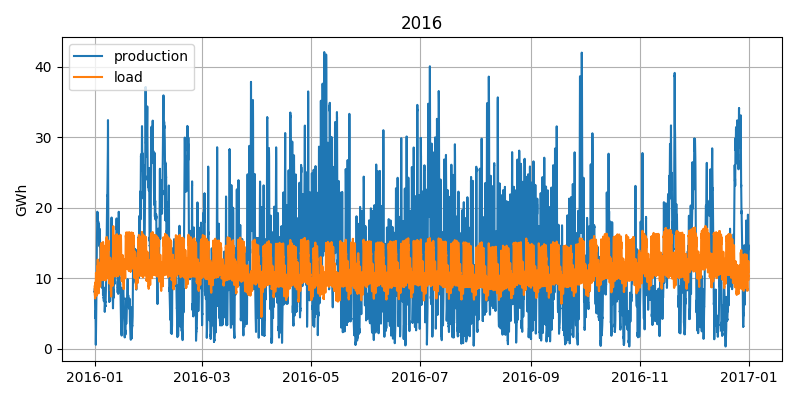As we might expect, we see a very constant and somewhat predictable load curve, while production varies wildly.

In :
fig = plt.figure(figsize=(8,4))
ax1 = plt.subplot()
x = generation.index
start = 1500
stop = start + 14*24*4
ax1.plot(x[start:stop],prod_fit[start:stop]/1E3,label="production")
ax1.set_ylabel("GWh")
plt.legend()
plt.grid()
plt.title("2016")
plt.ylim((0,36))
plt.tight_layout()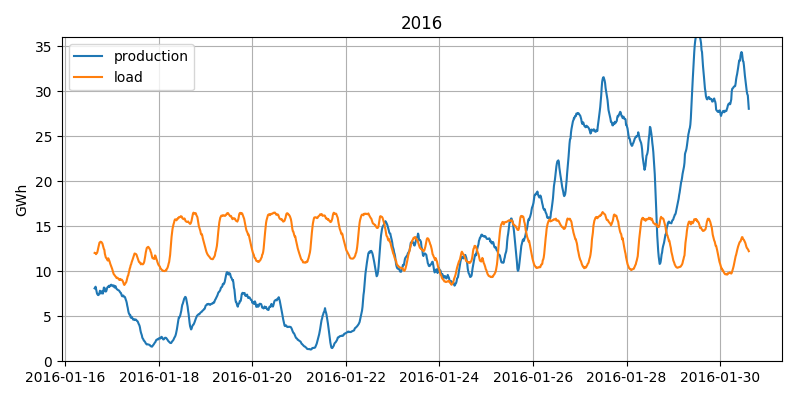If we zoom in on these two weeks in January, we can see one of the major problems to face already. During the first week we have almost constantly too little production, while in the second week we have excess production for most of the time. So we need to store the energy not only for a few hours (from mid day to provide light during the evening, for example), but for at least a full week.

So let's calculate how much we will store or draw from storage. We will ignore any losses at first, just for some very simple approximations. We just calculate the difference between production and consumption at any point in time:

$$D_t = P_t - C_t$$

We can then sum up this difference over time, which will give us the storage level at any point:

$$S_t = \sum_{i=0}^t P_i - C_i$$

Since we set $\sum_T P_t = \sum_T C_t$ we know that $S_t$ will be 0 for $t = T$ or, in other words, we will end with the same level in the storage as we begun. However, we might run below zero during the course of the year. To correct for that we just subtract the minumum point afterwards, as you will see.

In :
diff_15 = prod_fit - load_15  #our D_t
storage_15 = np.cumsum(diff_15)  #S_t

plt.figure(figsize=(8,4))
x = generation.index
ax1 = plt.subplot()
lines = ax1.plot(x,prod_fit/1E3,label="production")

ax1.set_ylabel("GWh")

ax2 = ax1.twinx()
#lines += ax2.plot(x,(storage_15)/1E6,'k--',label="storage")
lines += ax2.plot(x,(storage_15-min(storage_15))/1E6,'k',label="storage")
ax2.set_ylabel("TWh")
labels = [l.get_label() for l in lines]
plt.legend(lines,labels)
plt.title("2016")

plt.tight_layout()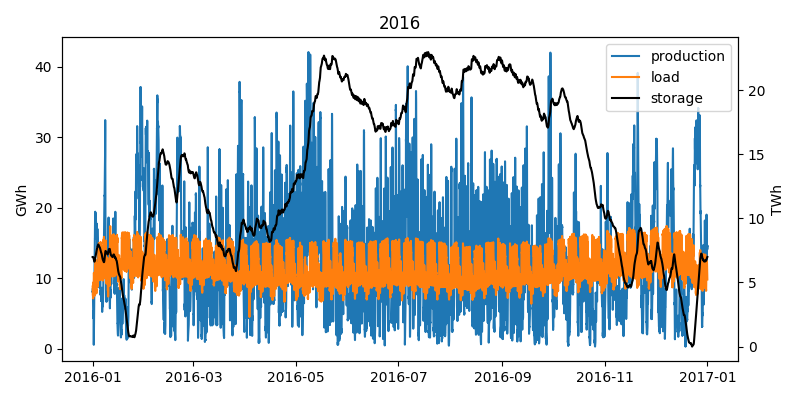It is important to note that we changed the scale of the storage axis (from GWh to TWh!), as otherwise we could not see anything.

So let's zoom in again into the two weeks of January.

In :
plt.figure(figsize=(8,4))
x = generation.index
start = 1500
stop = start + 14*24*4

ax1 = plt.subplot()
lines = ax1.plot(x[start:stop],prod_fit[start:stop]/1E3,label="production")

ax1.set_ylabel("GWh")
ax2.set_ylim((0,36))

ax2 = ax1.twinx()
#lines += ax2.plot(x,(storage_15)/1E6,'k--',label="storage")
lines += ax2.plot(x[start:stop],(storage_15[start:stop]-min(storage_15))/1E6,'k',label="storage")
ax2.set_ylabel("TWh")
ax2.set_ylim((0,8))
labels = [l.get_label() for l in lines]
plt.legend(lines,labels,loc=9)
plt.title("2016")

plt.tight_layout()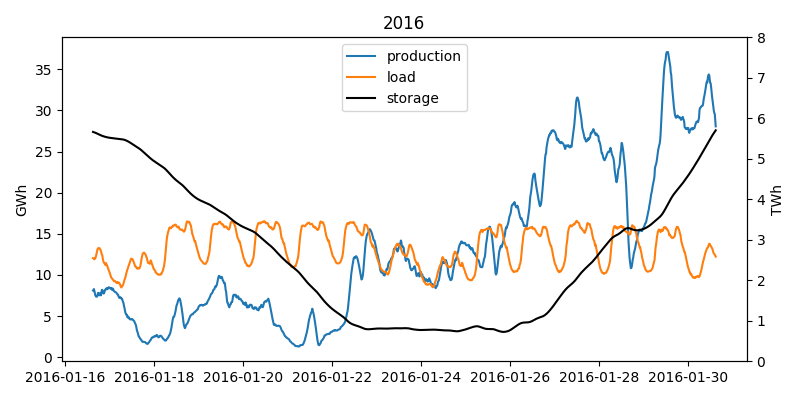As we would expect, storage gets drained during the first week and filled up again in the second. You might be wondering if the storage needed would change if we would include the beginning of the next and the end of the last year. Indeed we leave the storage the same way we found, however if the following year would require a higher starting level it would indeed change the outcome. However since we already analyzed a full year and each year is at least somewhat similar due to the seasons, including another week or minth should not change much about the estimation. The data is also available, so the analysis can be done, but for an estimate it‘s not really needed.

But how much storage do we need now?

In :
storage_needed_15 = (np.max(storage_15)-np.min(storage_15))
print("storage needed {:.2f} TWh".format(storage_needed_15/1E6 ))

storage needed 22.98 TWh


## Well ok 23 TWh, but how much is that?¶

Let's see: we can compare it to pumped hydro storage

In :
current_hydro = 37700  #MWh in Germany from Wikipedia
print("current pumped hydro: {} TWh\nupscale factor: {:.0f}".format(current_hydro/1E6,storage_needed_15/current_hydro))

current pumped hydro: 0.0377 TWh
upscale factor: 610


Ok, so we need more than 600x as much hydro as we currently have. So that does not seem like a good strategy.

Li-Ion batteries are all the rage these days, so why don‘t we use some of them? Lets see how many we would need.

In :
tesla_model_s = 0.1  #MWh
tesla_power_wall = 0.013 #MWh
tesla_power_pack = 0.2 #MWh
print("{:13,.0f} Model S batteries or\n{:13,.0f} power walls or\n{:13,.0f} power packs.".format(
storage_needed_15/tesla_model_s,storage_needed_15/tesla_power_wall,storage_needed_15/tesla_power_pack))

  229,833,410 Model S batteries or
1,767,949,310 power walls or
114,916,705 power packs.


So if we compare this with some of the well known Tesla products that use batteries we still find we a need a lot. In fact there are currently only about 43,000,000 cars in Germany. So even even they would all be Teslas and not used for driving, we would still need 4x as many cars.

Ok, but the production of batteries is picking up quickly around the world. So at least it should not take too long to make the batteries we need?

In :
battery_production_2016 = 28E3 # 28 gigawatt-hours
battery_production_2020 = 174E3 # 174 gigawatt-hours
print("With 2016 output it takes about {:.0f} years of battery production.".format(storage_needed_15/battery_production_2016))
print("With estimated 2020 output {:.0f} years.".format(storage_needed_15/battery_production_2020))

With 2016 output it takes about 821 years of battery production.
With estimated 2020 output 132 years.


That is too long, even if production doubles a few more times it takes at least 20 years and that would be for Germany only. We have too look into the details a bit more.

## Why do we even need so much?¶

In :
plt.figure(figsize=(8,4))
x = generation.index
start = 27600
stop = start + 34*24*4

ax1 = plt.subplot()
lines = ax1.plot(x[start:stop],prod_fit[start:stop]/1E3,label="production")

ax1.set_ylabel("GWh")
ax2.set_ylim((0,36))

ax2 = ax1.twinx()
#lines += ax2.plot(x,(storage_15)/1E6,'k--',label="storage")
lines += ax2.plot(x[start:stop],(storage_15[start:stop]-min(storage_15))/1E6,'k',label="storage (corrected)")
ax2.set_ylabel("TWh")
ax2.set_ylim((0,20))
labels = [l.get_label() for l in lines]
plt.legend(lines,labels)
plt.title("2016")

plt.tight_layout()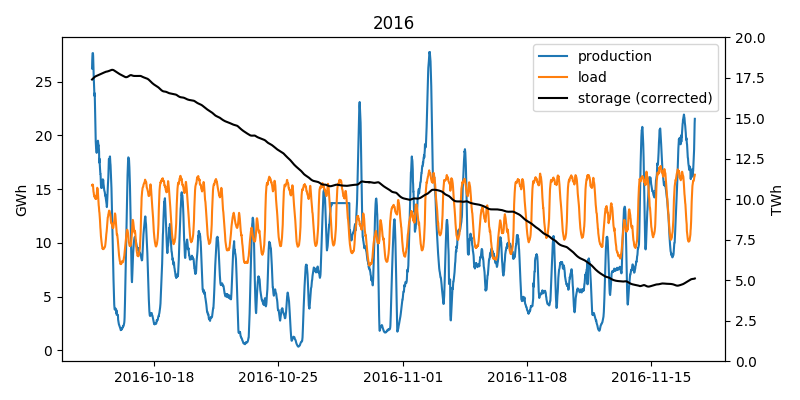If we look at the first plot showing the storage usage during the whole year, we can see how our storage gets filled during the summer months and then drains rapidly during autumn. As we can see in the above figure, from the middle of October till the middle of November we have almost constant under production. So much so that we would have drained almost 3/4 of our storage. These four weeks are the main reason we need so much storage.

## How to reduce the storage need?¶

Nevertheless, we are not underproducing all that much on most of the days. So what we could do is not just build as many renewables as we absolutely need, but just a few more. This will however complicate the storage calculation a bit.

What we have to do now is not just sum up the differences, as this would just fill our storage more and more. Instead we have to work our way backwards thought the year. For each timestep we check how much we over- or underproduced. If we have underproduction we add it to our storage need, since we now that this under production needs to be taken from storage which has to be filled in the days before. Then we look at the next time step which, since we are going backwards, is in fact the one before the current one. If we had over production in this time step, we can subtract it from you storage need until the storage need is 0. If we had underproduction in this timestep too we just add it to the storage need. We also keep track of the storage need for each time step we visit. This way if we need something from the storage in three consecutive days, we will have a very high storage need calculated in the day before that. But we also keep track of the possibility to refill the storage anytime.

In :
def calc_need(diff):
need = np.zeros_like(diff)
state = 0
for idx,d in enumerate(diff[::-1]):  #we iterate in reverse order
if state + d > 0:
need[idx] = 0
state = 0
else:
need[idx] = -state - d
state += d
return need[::-1]  #and then reverse the result again

In :
over_scale = 4.5
prod_over = prod_15 * over_scale

need = calc_need(diff_over)
storage_need = np.max(need)

print("storage needed {:.2f} TWh".format(storage_need/1E6))

storage needed 9.83 TWh


## With only a slight overproduction we already decreased the storage need quite dramatically, to about half of what we needed before.¶

We can also calculate how our storage level would look like at any given point in time and use it to check if our calculations are correct. We just set the maximum level of storage need we calculated by going backwards though the year as our storage capacity, then fill the storage with any over-production and drain it as needed.

Furthermore, with this kind of backtracking, we can also consider the storage losses that would occour from self-discharge of Li-Ion batteries. We just need to consider that our storage need grows every time we move one timestep. It grows since we are moving backwards through the year and we are keeping track of the storage needs. So during one step forward in time, we would loose some energy, which means if we go backwards we need more capacity.

In :
# Li-Ion batteries loose about 2-3% of charge per month of storage
qHour_in_month = 30*24*4
days_in_month = 30

def calc_need(diff,subs,loss_per_month = 1-0.02):
loss_per_sub = np.exp(np.log(loss_per_month)/subs)
need = np.zeros_like(diff)
state = 0
for idx,d in enumerate(diff[::-1]):
if state + d > 0:
need[idx] = 0
state = 0
else:
state /= loss_per_sub
need[idx] = -state - d
state += d
return need[::-1]

def calc_level(diff,subs,loss_per_month = 1-0.02):
loss_per_sub = np.exp(np.log(loss_per_month)/subs)
batt = np.zeros_like(diff)
tmp = -np.min(np.cumsum(diff))*1.1  # 1.1 as safety margin
for idx,d in enumerate(diff):
tmp = min((storage_need,tmp*loss_per_sub+d))
batt[idx] = tmp
return batt

In :
need = calc_need(diff_over,qHour_in_month)
storage_need = np.max(need)
level = calc_level(diff_over,qHour_in_month)

x = generation.index
plt.figure(figsize=(8,4))
plt.plot(x,level/1E6,label="level")
plt.plot(x,need/1E6,label="need")
plt.ylabel("TWh")
plt.grid()
plt.legend()
plt.tight_layout()